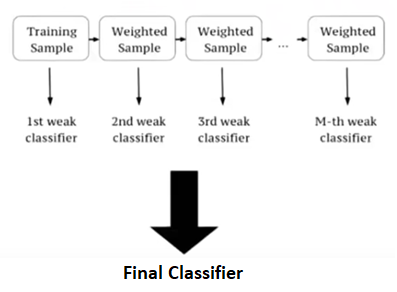Open in App
Not now

# ML | XGBoost (eXtreme Gradient Boosting)

• Last Updated : 09 Jun, 2022

XGBoost is an implementation of Gradient Boosted decision trees. This library was written in C++. It is a type of Software library that was designed basically to improve speed and model performance. It has recently been dominating in applied machine learning. XGBoost models majorly dominate in many Kaggle Competitions. In this algorithm, decision trees are created in sequential form. Weights play an important role in XGBoost. Weights are assigned to all the independent variables which are then fed into the decision tree which predicts results. The weight of variables predicted wrong by the tree is increased and the variables are then fed to the second decision tree. These individual classifiers/predictors then ensemble to give a strong and more precise model. It can work on regression, classification, ranking, and user-defined prediction problems.XGBoost Features The library is laser-focused on computational speed and model performance, as such, there are few frills. Model Features Three main forms of gradient boosting are supported:

System Features

• For use of a range of computing environments this library provides-
• Parallelization of tree construction
• Distributed Computing for training very large models
• Cache Optimization of data structures and algorithm

XGBoost enhancements/optimizations

XGBoost features various optimizations built-in to make the training faster when working with large datasets, in addition to its unique method of generating and pruning trees. Here is a handful of the most significant:

• Approximate Greedy Algorithm: instead of assessing every candidate split, this algorithm employs weighted quantiles to find the best node split.
• Cash-Aware Access: XGBoost stores data in the CPU’s cache memory.
• Sparsity: Aware Split Finding calculates Gain by putting observations with missing values onto the left leaf when there is some missing data. It then repeats the process by placing them in the appropriate leaf and selecting the scenario with the highest Gain.

Steps to Install Windows XGBoost uses Git submodules to manage dependencies. So when you clone the repo, remember to specify –recursive option:

`git clone --recursive https://github.com/dmlc/xgboost`

For Windows users who use Github tools, you can open the git-shell and type the following command:

```git submodule init
git submodule update```

OSX(Mac) First, obtain gcc-8 with Homebrew (https://brew.sh/) to enable multi-threading (i.e. using multiple CPU threads for training). The default Apple Clang compiler does not support OpenMP, so using the default compiler would have disabled multi-threading.

`brew install gcc@8`

Then install XGBoost with pip:

`pip3 install xgboost`

You might need to run the command with –user flag if you run into permission errors.

Example: Code: Python code for XGB Classifier

## Python3

 `# Importing the libraries` `from` `sklearn.metrics ``import` `confusion_matrix` `import` `xgboost as xgb` `from` `sklearn.model_selection ``import` `train_test_split` `from` `sklearn.preprocessing ``import` `LabelEncoder, OneHotEncoder` `import` `numpy as np` `import` `matplotlib.pyplot as plt` `import` `pandas as pd`   `# Importing the dataset` `dataset ``=` `pd.read_csv(``'Churn_Modelling.csv'``)` `X ``=` `dataset.iloc[:, ``3``:``13``].values` `y ``=` `dataset.iloc[:, ``13``].values`   `# Encoding categorical data` `labelencoder_X_1 ``=` `LabelEncoder()`   `X[:, ``1``] ``=` `labelencoder_X_1.fit_transform(X[:, ``1``])` `labelencoder_X_2 ``=` `LabelEncoder()`   `X[:, ``2``] ``=` `labelencoder_X_2.fit_transform(X[:, ``2``])` `onehotencoder ``=` `OneHotEncoder(categorical_features``=``[``1``])`   `X ``=` `onehotencoder.fit_transform(X).toarray()` `X ``=` `X[:, ``1``:]`   `# Splitting the dataset into the Training set and Test set` `X_train, X_test, y_train, y_test ``=` `train_test_split(` `    ``X, y, test_size``=``0.2``, random_state``=``0``)`   `# Fitting XGBoost to the training data` `my_model ``=` `xgb.XGBClassifier()` `my_model.fit(X_train, y_train)`   `# Predicting the Test set results` `y_pred ``=` `my_model.predict(X_test)`   `# Making the Confusion Matrix` `cm ``=` `confusion_matrix(y_test, y_pred)`

Output:

`Accuracy will be about 0.8645`

My Personal Notes arrow_drop_up
Related Articles